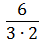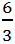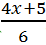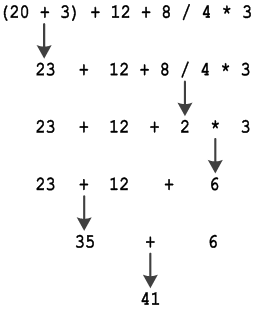Arithmetic operators follow the same precedence rules as in mathematics, and these are: exponentiation is performed first (when available), multiplication and division are performed next, addition and subtraction are performed last.

## PHP, Java, C++, C#

 Higher PrecedenceLower precedence Arithmetic Operators *, /, % +, –

## Visual Basic

 Higher PrecedenceLower precedence Arithmetic Operators ^ *, /, \, Mod +, –

## Python

 Higher PrecedenceLower precedence Arithmetic Operators ** *, /, //, % +, –

When multiplication and division exist in the same expression, and since both are of the same precedence, they are performed left to right (the same way as you read), which means that the expression

`6 / 3 * 2`

is equivalent to 4, (division is performed before multiplication).

If you want, however, the multiplication to be performed before the division, you can use parentheses to change the precedence, that is:

`6 / (3 * 2)`

which is equivalent toNotice: Computer programs can be written using your computer’s text editor application, but keep in mind that it is not possible to write fractions in the form ofor. Forget it! There is no equation editor in a text editor! All fractions must be written on one single line. For example,should be written as `6 / 3`, andshould be written as `(4 * x + 5) / 6`.

The order of operations can be summarized as follows:

1. Any operations enclosed in parentheses are performed first.
2. Any exponentiations (available in Visual Basic and Python) are performed next.
3. Then, any multiplication and division operations are performed from left to right.
4. In the end, any addition and subtraction operations are performed from left to right.

So, in the expression ` (20 + 3) + 12 + 8 / 4 * 3`

the operations are performed as follows:

1. 20 is added to 3, yielding a result of 23.
2. 8 is divided by 4, yielding a result of 2. This result is then multiplied by 3, yielding a result of 6.
3. 23 is added to 12, yielding a result of 35. This result is then added to 6, yielding a final result of 41.

Next is the same sequence of operations presented in a more graphical way.